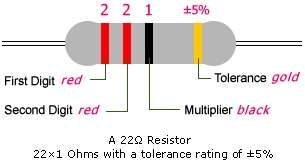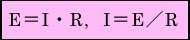# How to Read Resistor Color Codes?【Synton-Tech】

## How to Read Resistor Color Codes？

Resistor is one of the most common and widely used components in the electronics industry. For a resistor, our most intuitive understanding is how big the resistor is, that is, what is its resistance value

The reading methods of color ring resistance and chip resistance are respectively introduced below

First, from the bottom end of the resistor, find the color wheel that represents the tolerance accuracy. The gold color represents 5%, and the silver color represents 10%.

From the other end of the resistor, find the first and second color rings, and read the corresponding numbers. The following figure is an example. The first two color rings are red, so the corresponding numbers are red 2. 2

Then, read the third multiple color circle, black 1, so, in this example, the resistance value we get is 22ΩIf the third multiple color circle is gold, move the decimal point one place to the left. If the third multiple color circle is silver, move the decimal point two places to the left

【Color code to distinguish resistance value】【Resistance Range】

 SYMBOL A B C D F G J K M RESISTANCE TOLERANCE ±0.05% ±0.1% ±0.25% ±0.5% ±1% ±2% ±5% ±10% ±20%